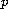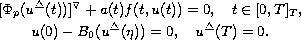Electron. J. Diff. Eqns., Vol. 2006(2006), No. 96, pp. 1-10.

### Existence, multiplicity and infinite solvability of positive solutions for p-Laplacian dynamic equations on time scales Da-Bin Wang

Abstract:
In this paper, by using Guo-Krasnosel'skii fixed point theorem in cones, we study the existence, multiplicity and infinite solvability of positive solutions for the following three-point boundary value problems for-Laplacian dynamic equations on time scalesBy multiplicity we mean the existence of arbitrary number of solutions.

Submitted April 14, 2006. Published August 22, 2006.
Math Subject Classifications: 34B10, 34B18, 39A10.
Key Words: Time scales; p-Laplacian; boundary value problem; positive solution; existence; multiplicity; infinite solvability.

Show me the PDF file (243K), TEX file, and other files for this article.Da-Bin Wang Department of Applied Mathematics Lanzhou University of Technology Lanzhou, Gansu, 730050, China email: wangdb@lut.cn